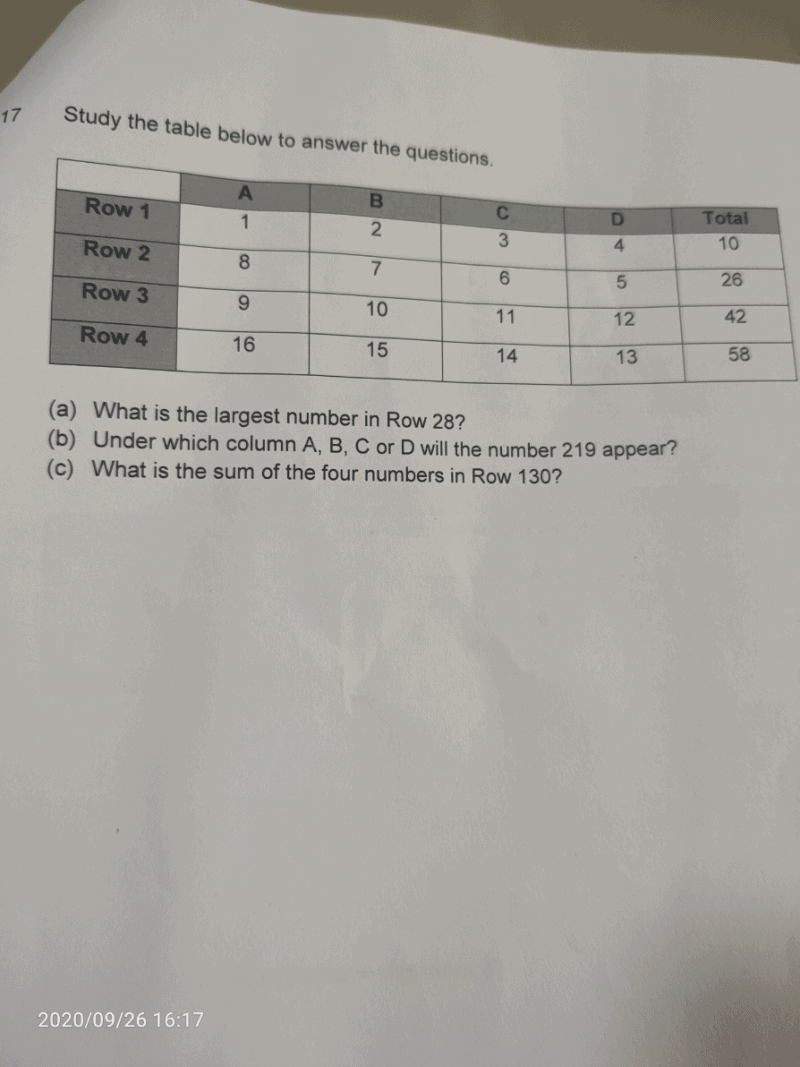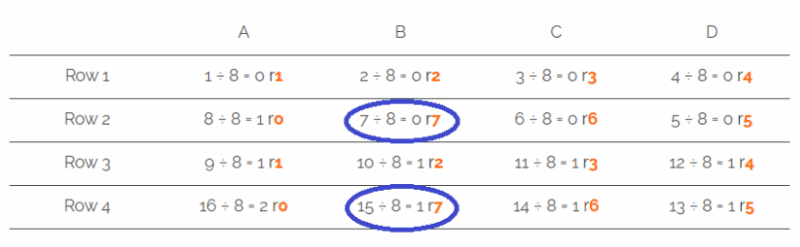# QuestionA B C D Row 1 1 ÷ 8 = 0 r1 2 ÷ 8 = 0 r2 3 ÷ 8 = 0 r3 4 ÷ 8 = 0 r4 Row 2 8 ÷ 8 = 1 r0 7 ÷ 8 = 0 r7 6 ÷ 8 = 0 r6 5 ÷ 8 = 0 r5 Row 3 9 ÷ 8 = 1 r1 10 ÷ 8 = 1 r2 11 ÷ 8 = 1 r3 12 ÷ 8 = 1 r4 Row 4 16 ÷ 8 = 2 r0 15 ÷ 8 = 1 r7 14 ÷ 8 = 1 r6 13 ÷ 8 = 1 r5

The trick to this kind of question is to look at the remainders.

As for what number to divide the number in the cell by, it is determined by the number of terms in the sequence. In this case, there are 8 terms before the sequence repeats.

(a) Largest number in an even row is at column A.

The value of A-even can be found by (Row number ÷ 2) × 8.

Hence, the largest number in Row 28 is (28 ÷ 2) × 8 = 112

(note: I don’t think they are looking for the total for Row 28. Else, it will just be the same question as (c))

(b) 219 ÷ 8 = 27 r3

Column C

(c)

Again, largest number on the even Row 130 is at A -> (130 ÷ 2) × 8 = 520

So the 4 numbers are

A 520, B 519, C 518, D 517

Total = 2074